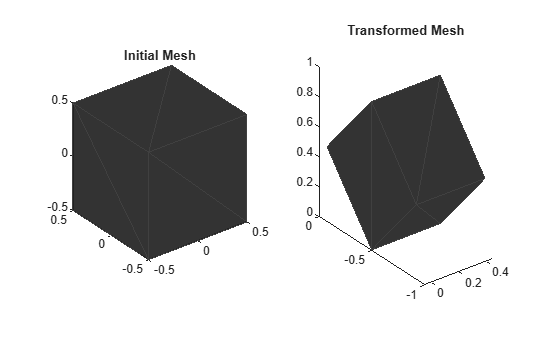# applyTransform

Apply forward transformation to mesh vertices

## Syntax

``transformedMesh = applyTransform(mesh,T)``

## Description

example

````transformedMesh = applyTransform(mesh,T)` applies the forward transformation matrix `T` to the vertices of the object mesh.```

## Examples

collapse all

Create an `extendedObjectMesh` object and transform the object by using a transformation matrix.

Create a cuboid mesh of unit dimensions.

`cuboid = extendedObjectMesh('cuboid');`

Create a transformation matrix that is a combination of a translation, a scaling, and a rotation.

```tform = makehgtform('translate',[0.2 -0.5 0.5], ... 'scale',[0.5 0.6 0.7], ... 'xrotate',pi/4);```

Transform the mesh.

`transformedCuboid = applyTransform(cuboid,tform);`

Visualize the meshes.

```subplot(1,2,1); show(cuboid); title('Initial Mesh') subplot(1,2,2); show(transformedCuboid); title('Transformed Mesh')```## Input Arguments

collapse all

Extended object mesh, specified as an `extendedObjectMesh` object.

Transformation matrix applied on the object mesh, specified as a 4-by-4 matrix. The 3-D coordinates of each point in the object mesh is transformed according to this formula:

`[xT; yT; zT; 1] = T*[x; y; z; 1]`

`xT`, `yT`, and `zT` are the transformed 3-D coordinates of the point.

Data Types: `single` | `double`

## Output Arguments

collapse all

Transformed object mesh, returned as an `extendedObjectMesh` object.

## Version History

Introduced in R2020b